Q-Chem 5.1 User’s Manual

# 5.10 Asymptotically Corrected Exchange-Correlation Potentials

No GGA exchange functional can simultaneously produce the correct contribution to the exchange energy density and exchange potential in the asymptotic region of molecular systems, and existing GGA exchange-correlation (XC) potentials decay much faster than the correct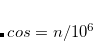XC potential in the asymptotic region. High-lying occupied orbitals and low-lying virtual orbitals are therefore too loosely bound, and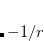becomes far smaller than the ionization energy, despite the exact condition that these should be the same for the exact functional. Moreover, response properties may be poorly predicted from TDDFT calculations with GGA functionals. Long-range corrected hybrid DFT (LRC-DFT), described in Section 5.6, has greatly remedied this situation, but is more expensive that KS-DFT with GGA functionals due to the use of Hatree-Fock exchange. The asymptotic corrections described in this section are designed to remedy the same problems but within the GGA framework.

## 5.10.1 LB94 Scheme

An asymptotically corrected (AC) exchange potential proposed by van Leeuwen and Baerends is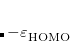(5.64)

whereis the reduced density gradient. For an exponentially-decaying density, this potential reduces toin the asymptotic region of molecular systems. The LB94 xc potential is formed by a linear combination of LDA XC potential and the LB exchange potential: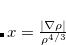(5.65)

The parameter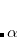in Eq. eq:vx_LB was determined by fitting to the exact XC potential for Be atom. As mentioned in Refs. Casida:1998 and Hirata:1999b, for TDDFT calculations, it is sufficient to include the AC XC potential for ground-state calculations followed by TDDFT calculations with an adiabatic LDA XC kernel. The implementation of the LB94 XC potential in Q-Chem takes this approach, using the LB94 XC potential for the ground state calculations, followed by a TDDFT calculation with an adiabatic LDA XC kernel. This TDLDA/LB94 approach has been widely applied to study excited-state properties of large molecules.

Since the LB exchange potential in Eq. eq:vx_LB does not come from the functional derivative of an exchange energy functional, the Levy-Perdew virial relation is used instead to obtain the exchange energy: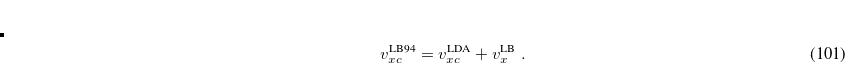(5.66)

An LB94 calculation is requested by setting EXCHANGE = LB94 in the $rem section. Additional job control and examples appear below. LB94_BETA  Sets theparameter for the LB94 XC potential TYPE:  INTEGER DEFAULT:  500 OPTIONS: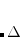Corresponding to. RECOMMENDATION:  Use the default. Example 5.60 Applications of LB94 XC potential to N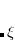molecule. $comment
TDLDA/LB94 calculation is performed for excitation energies.
$end$molecule
0 1
N    0.0000    0.0000    0.0000
N    1.0977    0.0000    0.0000
$end$rem
JOBTYPE     = sp
EXCHANGE    = lb94
BASIS       = 6-311(2+,2+)G**
CIS_N_ROOTS = 30
RPA         = true
$end  ## 5.10.2 Localized Fermi-Amaldi (LFA) Schemes Another alternative, proposed by Pan, Fang and Chai, is to use a localized version of Fermi-Amaldi exchange-correlation functional. The resulting exchange density functional, whose functional derivative has the correctasymptotic behavior, can be directly added to any semi-local density functional. Three variants of this method were proposed in Ref. Chai:2013b. The simplest of these, the strictly-localized Fermi-Amaldi (LFAs) scheme, is implemented in Q-Chem, for molecules consisting of atoms with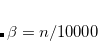. Example 5.61 LFAs-PBE single-point TD-DFT calculation with water molecule $comment
Use LFAs-PBE potential for ground-state calculations, followed by
TDDFT calculations with an adiabatic PBE XC kernel.
$end$molecule
0  1
O
H1  O  oh
H2  O  oh  H1  hoh

oh  =   1.0
hoh = 110.0
$end.$rem
JOBTYPE       sp
EXCHANGE      gen
BASIS         6-311(2+,2+)G**
CIS_N_ROOTS   30
RPA           true
$end$xc_functional
X   PBE   1.0
C   PBE   1.0
X   LFAs  1.0
\$end